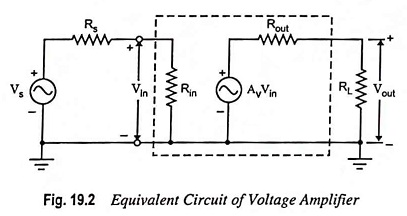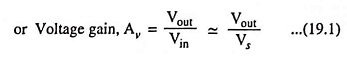## Voltage Amplifier – Definition and Equivalent Circuit:

Voltage amplifiers are intended to amplify an input voltage signal and provide an output voltage signal. The voltage amplifiers is essentially a voltage-controlled voltage source. The input impedance is required to be high and output impedance is required to be low. Since the signal source is essentially a voltage source, it is convenient to represent it in terms of a Thevenin equivalent circuit (Fig. 19.2). In a voltage amplifier the output quantity of interest is the output voltage.From the equivalent circuit of a voltage amplifiers depicted in Fig. 19.2, we find that if the input resistance Rin >> Rs then Vin ≈ Vs and if load resistance RL >> Rout, then Vout ≈ AvVinAn ideal voltage amplifiers should have infinite input resistance and zero output resistance.

The amplifier provides a voltage output proportional to the voltage input and the proportionality constant is independent of the magnitudes of source and load resistances.

Scroll to Top